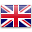# SAM Space (mind-map style)

Sort by:
##### 1.1 光和陰影 Light and Shadows

STEM lessons + blocks

Students will gain understanding of how light and...##### 1.10 互動故事書 Interactive Storybook

STEM lessons + blocks

2. Students will write a creative story and...##### 1.11 SAM保險櫃 SAM Safe

STEM lessons + blocks

Students will learn about algorithms and boolean (if/then)...##### 1.2 創作一首歌 Compose a Song

STEM lessons + blocks

Students will gain an understanding of music therapy...##### 1.3 設計一個棲息地 Design a Habitat

STEM lessons + blocks

Students will gain understanding of how living things...##### 1.4 智能照明系統 Smart Lighting Systems

STEM lessons + blocks

Students will gain an understanding of what ‘Smart...##### 1.5 時間到了! Time's Up!

STEM lessons + blocks

Students will solve word problems involving addition and...##### 1.6 房子牢固性測試 Build it up, break it down

STEM lessons + blocks

Students will investigate how a house can be...##### 1.7 種子的傳播 Seed Dispersal

STEM lessons + blocks

Students will gain understanding of seed dispersal as...STEM lessons + blocks

Students will gain understanding of how technology can...##### 1.9 減廢, 重用, 循環再造 Reduce, Reuse, Recycle

STEM lessons + blocks

Students will learn to recycle, working collaboratively to...##### 2.1 小夜燈 Night Light

STEM lessons + blocks

Students will gain understanding of how a product...##### 2.10 質數與合成數 There can be Only, Two

STEM lessons + blocks

Students will use their knowledge of prime numbers,...##### 2.2 音樂盒 Music Box

STEM lessons + blocks

Students will gain understanding of how systems and...##### 2.3 播種生長 Sow and Grow

STEM lessons + blocks

Students will gain understanding of how change occurs...##### 2.4 阻力與摩擦力 Resistance and Friction

STEM lessons + blocks

Students learn the concept of friction and the...##### 2.5 智能門鈴 Smart Door Bell

STEM lessons + blocks

Students will look at the use of sensors...##### 2.6 磁力 Magnetic Force

STEM lessons + blocks

Students will work collaboratively to investigate cause and...##### 2.7 打鼓模擬器 Drum Simulator

STEM lessons + blocks

Students will gain understanding of how the speed...##### 2.8 侵蝕 Erosion

STEM lessons + blocks

Students will gain understanding of water erosion and...##### 2.9 配對數字 Paired Up Numbers

STEM lessons + blocks

Students will investigate the factors of numbers with...##### 3.1 物質的性質 Properties of Matter

STEM lessons + blocks

Students will gain understanding of how an object...##### 3.11 撇號 Apostrophe

STEM lessons + blocks

Students will learn how an apostrophe is used....##### 3.2 摩斯密碼 Morse Code

STEM lessons + blocks

Students will learn how Morse Code enabled efficient,...##### 3.3 結他 Guitar

STEM lessons + blocks

Students will gain understanding of how stringed instruments...##### 3.4 地震模擬器 Earthquake Simulator

STEM lessons + blocks

Students will gain understanding of what an earthquake...##### 3.5 火星探測號 Mars Rover

STEM lessons + blocks

Students will identify two possible solutions for designing...##### 3.6 燈塔 Lighthouse

STEM lessons + blocks

Students will investigate how light reflecting from objects...##### 3.7 信息的傳遞 SAM Insect

STEM lessons + blocks

Students will work collaboratively to investigate how information...##### 3.8 熔岩燈 SAM Lava Lamp

STEM lessons + blocks

Students will gain understanding of water erosion and...##### 4.1 探索電路 Exploring Circuit

STEM lessons + blocks

Students will gain understanding of how electricity is...##### 4.10 比率 Ratio

STEM lessons + blocks

Students will work with collections of physical objects,...##### 4.2 地球和軌跡 Earth and Orbit

STEM lessons + blocks

Students will gain understanding of what an orbit...##### 4.3 黑夜與白晝 Night and Day

STEM lessons + blocks

Students will gain understanding of how the Earth...##### 4.4 摩斯密碼警報 Morse Code Alert

STEM lessons + blocks

Students will extend their knowledge of Morse Code,...##### 4.5 密碼與模式 Password and Patterns

STEM lessons + blocks

In the first session, students will...##### 4.6 牛頓第三運動定律 Every Action ....

STEM lessons + blocks

Students will gain understanding of Newton’s Third Law...##### 4.7 探索遺傳 Exploring Heredity

STEM lessons + blocks

Students will work collaboratively to investigate heredity and...##### 4.9 飲水機監控系統 Water Dispenser

STEM lessons + blocks

Students will gain understanding of the positive and...